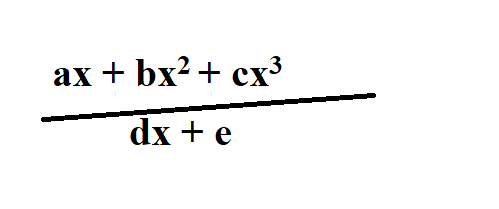# Summary Notes for Polynomials and Partial FractionsHere are summary for polynomials (factor, remainder theorem, and solving cubic equations):

## Remainder Theorem:

For a polynomial P(x), when P(x) is divided by (x -b), the remainder is P(b).

## Factor Theorem:

For a polynomial P(x), if (x-b) is a factor of P(x), then P(b) = 0 (since remainder = 0).

## Solving cubic equations (for O Level Additional Mathematics):

The O level method of solving cubic equation involves transforming the cubic equation ax3 + bx2 + cx + d =0 in (x- g)(x-h)(x-f) = 0. Then the solutions of x are gh and f

## Partial Fractions

In O Level Additional Mathematics, students are required to decompose a fraction with denominator of (ax+b)(cx+d) or (ax+b)2(cx+d) or (ax+b)(cx2+d) into partial fractions.

To do so, students need to first make the fraction into proper fractions, then find the unknowns based on identities.

## Want to learn O Level Add Maths on Demand?

In my course on O Level Additional Mathematics, I go into detail not only the concepts but how to apply them in different scenarios. We will go through many exam questions together step-by-step so that students finish the course equipped with the skills needed to tackle their test and exam questions. You can purchase the course instantly here, and watch and learn instantly.

error: Content is protected !!